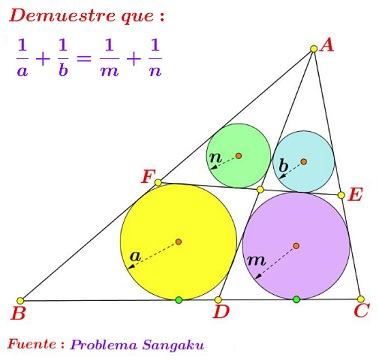# Sangaku via Peru

### Source### Solution

Let $G\;$ be the point of intersection of $AD\;$ and $EF.\;$ Denote $GE=a_1,\;$ $GF=a_2,\;$ the semiperimeters of triangles $AGE\;$ and $AGF\;$ $p_1\;$ and $p_2,\;$ respectively; $[\Delta AGE]=S_1,\;$ $[\Delta AGF]=S_2.$

The circle $(CDGE)\;$ is $A-$excircle of $\Delta AGE,\;$ implying $\displaystyle\frac{1}{m}=\frac{p_1-a_1}{S_1}.\;$ Similarly, from $(BDGF)\;$ being an excircle of $\Delta AGF,\;$ $\displaystyle\frac{1}{a}=\frac{p_2-a_2}{S_2}.\;$ Clearly, $\displaystyle\frac{1}{b}=\frac{p_1}{S_1}\;$ and $\displaystyle\frac{1}{n}=\frac{p_2}{S_2},\;$ so that $\displaystyle\frac{1}{a}+\frac{1}{b}=\frac{1}{m}+\frac{1}{n}\;$ is equivalent to

$\displaystyle\frac{p_2-a_2}{S_2}+\frac{p_1}{S_1}=\frac{p_1-a_1}{S_1}+\frac{p_2}{S_2},$

which in turn is equivalent to $\displaystyle\frac{a_1}{a_2}=\frac{S_1}{S_2}\;$ which is true.

### Acknowledgment

Leo Giugiuc has kindly posted the above problem, with his solution, at the CutTheKnotMath face book page. Leo cam across the problem at a Miguel Ochoa Sanchez's post at the PERU GEOMÉTRICO facebook group.

• Sangaku: Reflections on the Phenomenon
• Critique of My View and a Response
• 1 + 27 = 12 + 16 Sangaku
• 3-4-5 Triangle by a Kid
• 7 = 2 + 5 Sangaku
• A 49th Degree Challenge
• A Geometric Mean Sangaku
• A Hard but Important Sangaku
• A Restored Sangaku Problem
• A Sangaku: Two Unrelated Circles
• A Sangaku by a Teen
• A Sangaku Follow-Up on an Archimedes' Lemma
• A Sangaku with an Egyptian Attachment
• A Sangaku with Many Circles and Some
• A Sushi Morsel
• An Old Japanese Theorem
• Archimedes Twins in the Edo Period
• Arithmetic Mean Sangaku
• Bottema Shatters Japan's Seclusion
• Chain of Circles on a Chord
• Circles and Semicircles in Rectangle
• Circles in a Circular Segment
• Circles Lined on the Legs of a Right Triangle
• Equal Incircles Theorem
• Equilateral Triangle, Straight Line and Tangent Circles
• Equilateral Triangles and Incircles in a Square
• Five Incircles in a Square
• Four Hinged Squares
• Four Incircles in Equilateral Triangle
• Gion Shrine Problem
• Harmonic Mean Sangaku
• Heron's Problem
• In the Wasan Spirit
• Incenters in Cyclic Quadrilateral
• Japanese Art and Mathematics
• Malfatti's Problem
• Maximal Properties of the Pythagorean Relation
• Neuberg Sangaku
• Out of Pentagon Sangaku
• Peacock Tail Sangaku
• Pentagon Proportions Sangaku
• Proportions in Square
• Pythagoras and Vecten Break Japan's Isolation
• Radius of a Circle by Paper Folding
• Review of Sacred Mathematics
• Sangaku à la V. Thebault
• Sangaku and The Egyptian Triangle
• Sangaku in a Square
• Sangaku Iterations, Is it Wasan?
• Sangaku with 8 Circles
• Sangaku with Angle between a Tangent and a Chord
• Sangaku with Quadratic Optimization
• Sangaku with Three Mixtilinear Circles
• Sangaku with Versines
• Sangakus with a Mixtilinear Circle
• Sequences of Touching Circles
• Square and Circle in a Gothic Cupola
• Steiner's Sangaku
• Tangent Circles and an Isosceles Triangle
• The Squinting Eyes Theorem
• Three Incircles In a Right Triangle
• Three Squares and Two Ellipses
• Three Tangent Circles Sangaku
• Triangles, Squares and Areas from Temple Geometry
• Two Arbelos, Two Chains
• Two Circles in an Angle
• Two Sangaku with Equal Incircles
• Another Sangaku in Square
• Sangaku via Peru
• FJG Capitan's Sangaku Copyright © 1996-2018 Alexander Bogomolny
• 69981653